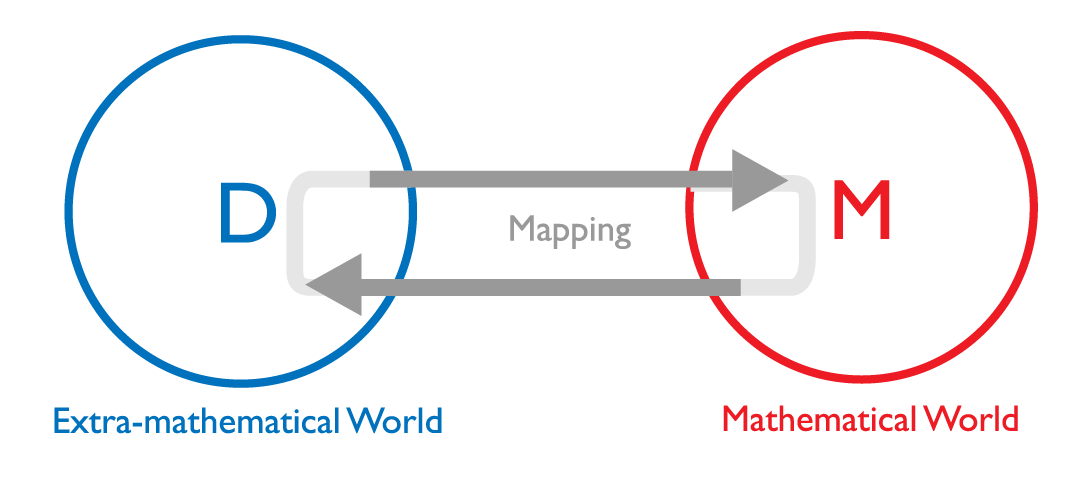# What is mathematical modelling?

Mathematical modelling is:

• a process in which real-life situations and relations in these situations are expressed by using mathematics (Haines and Crouch, 2007), or;
• a cyclical process in which real-life problems are translated into mathematical language, solved within a symbolic system, and the solutions tested back within the real-life system (Verschaffel, Greer, and De Corte, 2002).

In both instances, mathematical models are seen to move beyond the physical characteristics of a real-life situation to examine its structural features through mathematics; it entails the construction of mathematical models of natural and social phenomena that are problem-driven, and where the choice of relevant mathematics is itself part of the solving process.

From a theoretical point of view, the concept of mathematical modelling can be represented in three parts (Fig.1):

1. an extra-mathematical domain (D)
2. some mathematical domain (M)
3. mapping from the extra-mathematical domain (D) to the mathematical domain (M), resulting in outcomes that are translated back to the extra-mathematical domain (D).

Figure 1.The ‘extra-mathematical’ domain (D) is a useful way of representing the ‘real world’ that is relevant to a particular problem.

Objects, relations, phenomena, assumptions, questions, etc. in (D) are identified and selected as relevant for purpose or situation and are then ‘mapped’ – translated –  into relations, phenomena, assumptions, questions, etc. pertaining to the mathematical domain (M).

Within (M) mathematical deliberations, manipulations and inferences are made, the outcomes of which are then translated back to D and interpreted as conclusions concerning that domain.

In this activity the use of technology is relevant whenever it can enhance the mathematical process – which is often.

This modelling cycle may be iterated several times on the basis of validation and evaluation of the model in relation to the domain, until the resulting conclusions are satisfactory in relation to the purpose of the model construction.

Reference: Niss, M., Blum, W., & Galbraith, P. (2007). Introduction. In W. Blum, P. Galbraith, M. Niss, & H-W. Henn (Eds.), Modelling and Applications in Mathematics Education: The 14th ICMI Study, (pp. 3–32). New York: Springer.